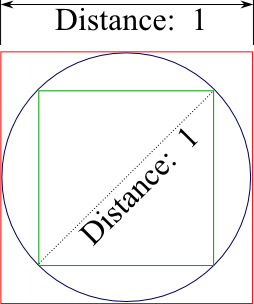# Pi - Importance and calculation

$$\pi$$ (Pi /paɪ/) is known as a magical number in mathematics. Is is the ratio of a circle’s circumference to its diameter, and is commonly approximated as 3.14159.

### Importance

• It seems a simple enough concept. But it turns out to be an “irrational number,” meaning its exact value is inherently unknowable. Computer scientists have calculated billions of digits of pi, starting with 3.14159265358979323…, but because no recognizable pattern emerges in the succession of its digits, we could continue calculating the next digit, and the next, and the next, for millennia, and we’d still have no idea which digit might emerge next. The digits of pi continue their senseless procession all the way to infinity.
• Ancient mathematicians apparently found the concept of irrationality completely maddening. It struck them as an affront to the omniscience of God, for how could the Almighty know everything if numbers exist that are inherently unknowable?
• Pi’s ubiquity goes beyond math. The number crops up in the natural world, too. It appears everywhere there’s a circle, of course, such as the disk of the sun, the spiral of the DNA double helix, the pupil of the eye, the concentric rings that travel outward from splashes in ponds. Pi also appears in the physics that describes waves, such as ripples of light and sound. It even enters into the equation that defines how precisely we can know the state of the universe, known as Heisenberg’s uncertainty principle.
• Pi emerges in the shapes of rivers. A river’s windiness is determined by its “meandering ratio,” or the ratio of the river’s actual length to the distance from its source to its mouth as the crow flies. Rivers that flow straight from source to mouth have small meandering ratios, while ones that lollygag along the way have high ones. Turns out, the average meandering ratio of rivers approaches — you guessed it — pi.

### Approximation

• We can measure $$\pi$$ by using a physical wheel as shown in the above animation, but we can’t get more than a digit or two of accuracy.π - Another method to measure Pi is by bracketing a circle with polygons. - There are [many formulae](https://www.wikiwand.com/en/Approximations_of_%CF%80#/Development_of_efficient_formulae) that have been developed over the course of all these years to obtain an efficient approximation of our magical number, $$\pi$$.